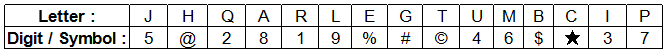# SBI Clerk 06 July 2008

Instructions

Study the following information carefully and answer the questions given below :

M, D, J, Q, T, F, H and N are sitting around a circle facing at the centre. T is third to the right of F who is second to the left of M. Q is not a neighbour of T or F and is third to the left of H. J is second to the right of N.

Question 181

Question 182

Question 183

Question 184

Question 185

# Who is second to the right of T ?

Instructions

In each question below is given a group of letters followed by four combinations of digits/symbols numbered a, b, c and d. You have to find out which of the combinations correctly represents the group of letters based on the following coding system and mark the number of that combination as the answer. If none of the four combinations correctly represents the group of letters, mark e: i.e. ‘None of these’ as the answer. SymbolConditions :
(i) If both the first and the last letters of the group are vowels, their codes are to be interchanged.
(ii) If the first letter is a consonant and the last letter is a vowel, both are to be coded by the code for the consonant.

Question 186

Question 187

Question 188

Question 189

Question 190

OR